# PROGRAMMING IN HASKELL Odds and Ends and Type

• Slides: 51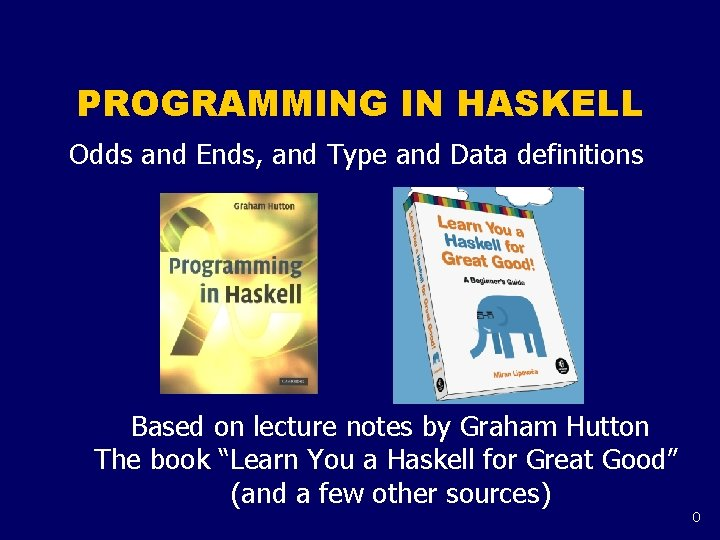PROGRAMMING IN HASKELL Odds and Ends, and Type and Data definitions Based on lecture notes by Graham Hutton The book “Learn You a Haskell for Great Good” (and a few other sources) 0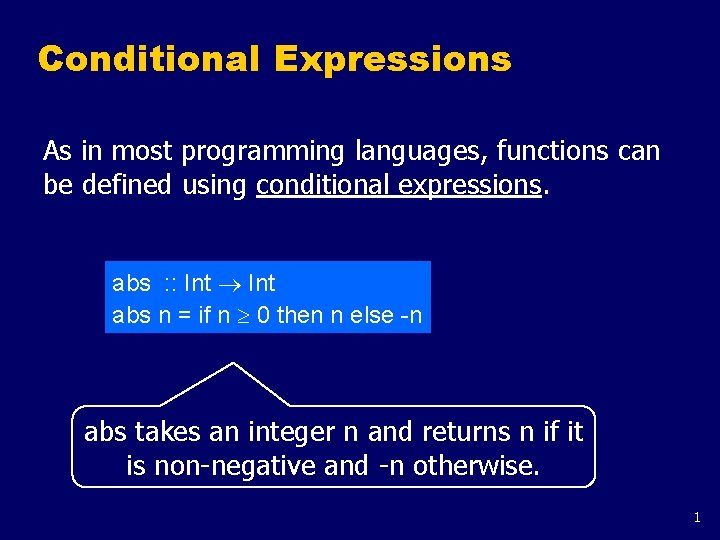Conditional Expressions As in most programming languages, functions can be defined using conditional expressions. abs : : Int abs n = if n 0 then n else -n abs takes an integer n and returns n if it is non-negative and -n otherwise. 1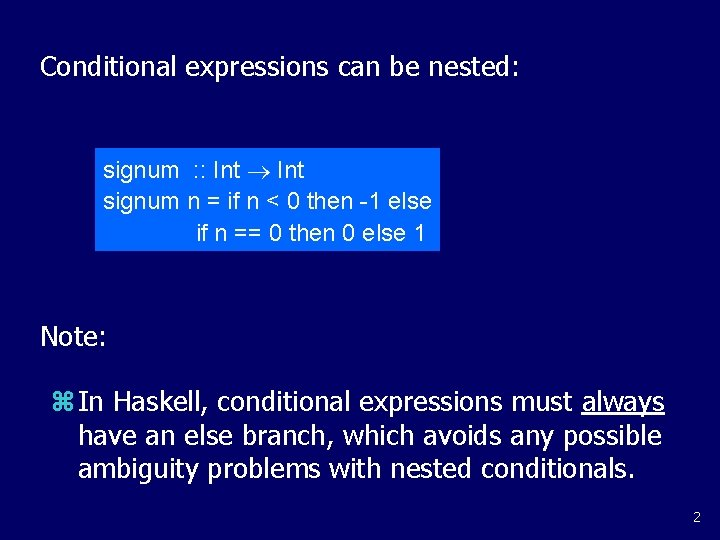Conditional expressions can be nested: signum : : Int signum n = if n < 0 then -1 else if n == 0 then 0 else 1 Note: z In Haskell, conditional expressions must always have an else branch, which avoids any possible ambiguity problems with nested conditionals. 2Guarded Equations As an alternative to conditionals, functions can also be defined using guarded equations. abs n | n 0 = n | otherwise = -n As previously, but using guarded equations. 3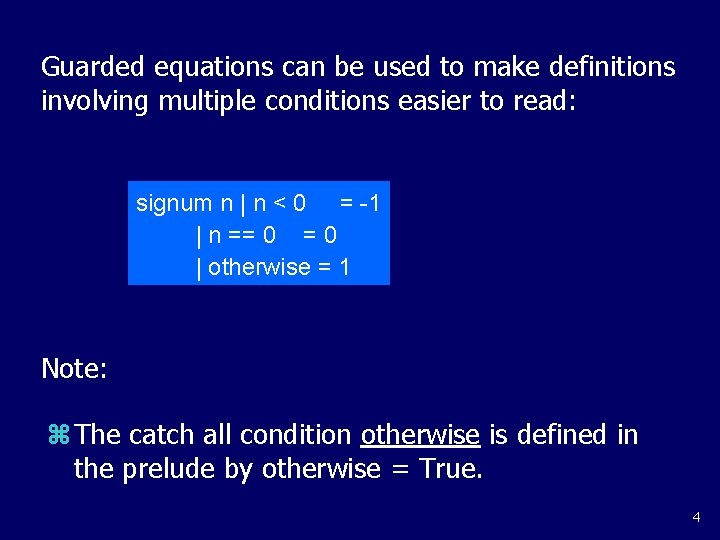Guarded equations can be used to make definitions involving multiple conditions easier to read: signum n | n < 0 = -1 | n == 0 | otherwise = 1 Note: z The catch all condition otherwise is defined in the prelude by otherwise = True. 4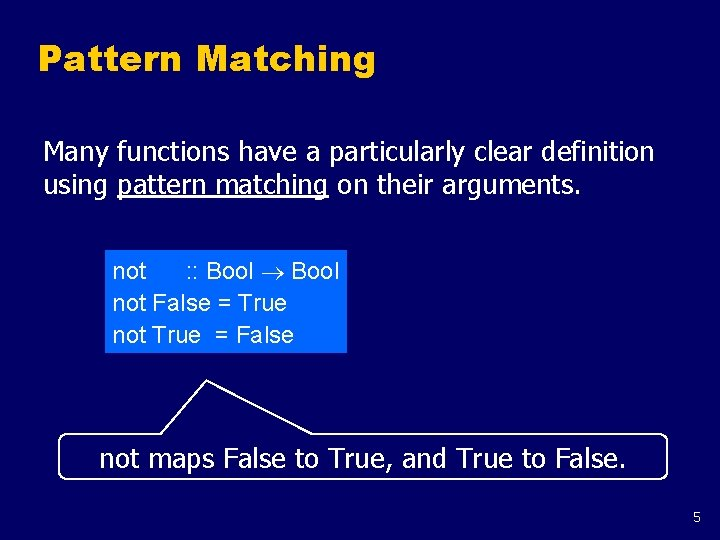Pattern Matching Many functions have a particularly clear definition using pattern matching on their arguments. not : : Bool not False = True not True = False not maps False to True, and True to False. 5Functions can often be defined in many different ways using pattern matching. For example (&&) : : Bool True && True = True && False = False && True = False && False = False can be defined more compactly by True && True = True _ && _ = False 6However, the following definition is more efficient, because it avoids evaluating the second argument if the first argument is False: True && b = b False && _ = False Note: z The underscore symbol _ is a wildcard pattern that matches any argument value. 7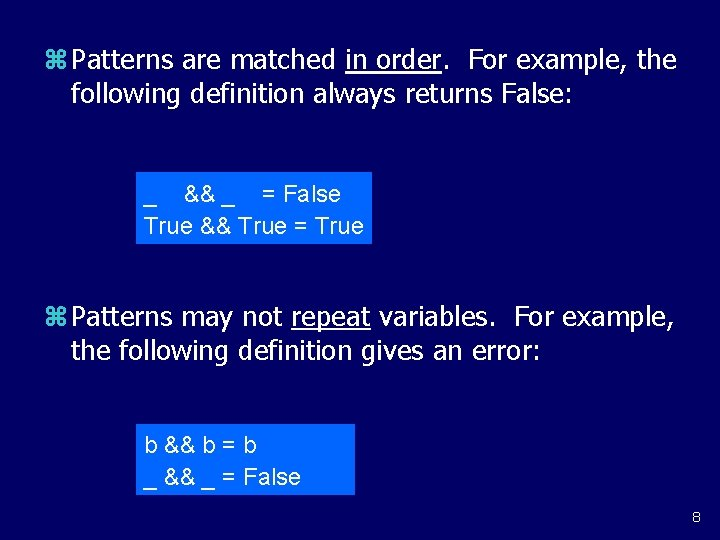z Patterns are matched in order. For example, the following definition always returns False: _ && _ = False True && True = True z Patterns may not repeat variables. For example, the following definition gives an error: b && b = b _ && _ = False 8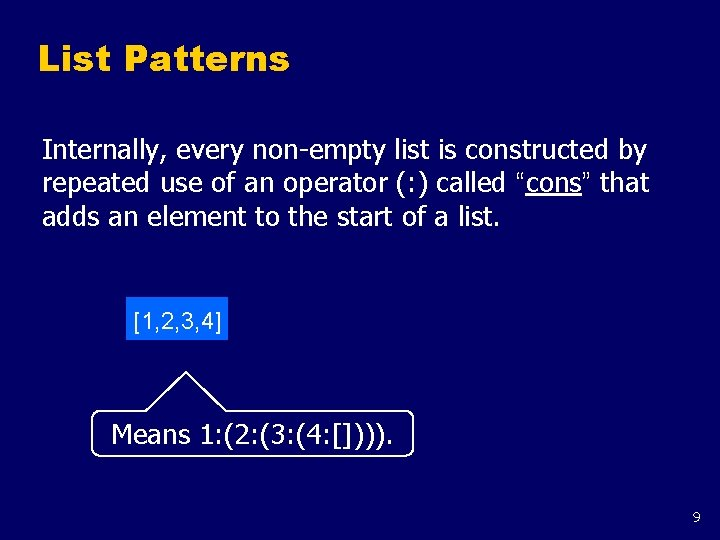List Patterns Internally, every non-empty list is constructed by repeated use of an operator (: ) called “cons” that adds an element to the start of a list. [1, 2, 3, 4] Means 1: (2: (3: (4: []))). 9Functions on lists can be defined using x: xs patterns. head : : [a] a head (x: _) = x tail : : [a] tail (_: xs) = xs head and tail map any non-empty list to its first and remaining elements. 10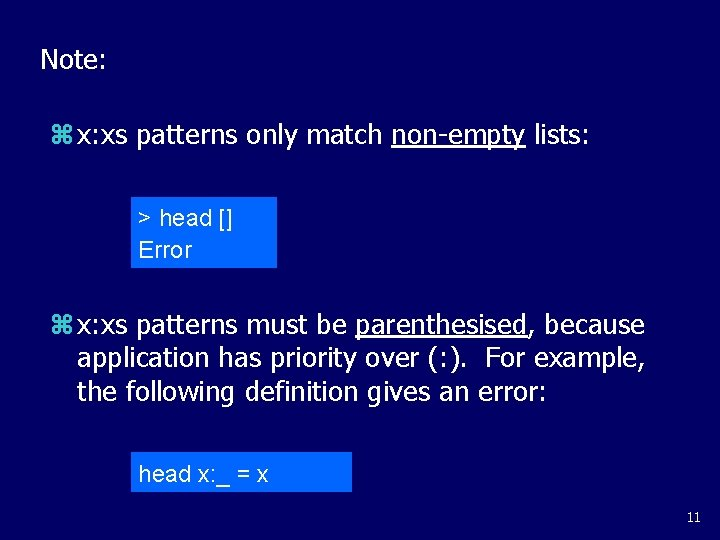Note: z x: xs patterns only match non-empty lists: > head [] Error z x: xs patterns must be parenthesised, because application has priority over (: ). For example, the following definition gives an error: head x: _ = x 11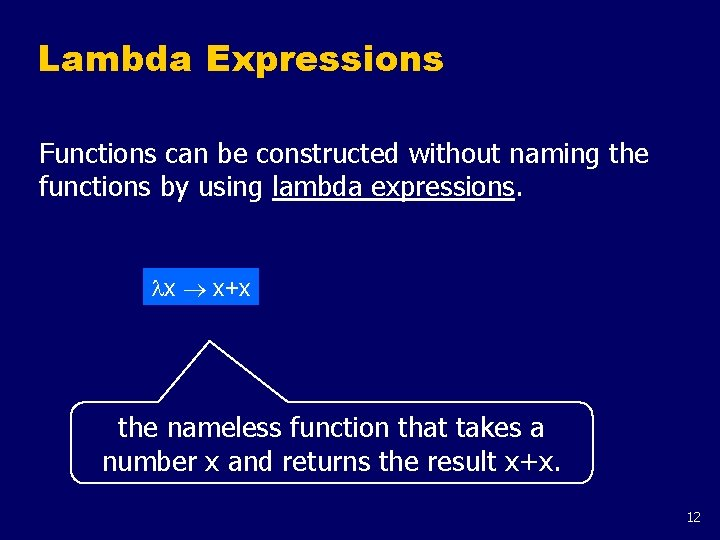Lambda Expressions Functions can be constructed without naming the functions by using lambda expressions. x x+x the nameless function that takes a number x and returns the result x+x. 12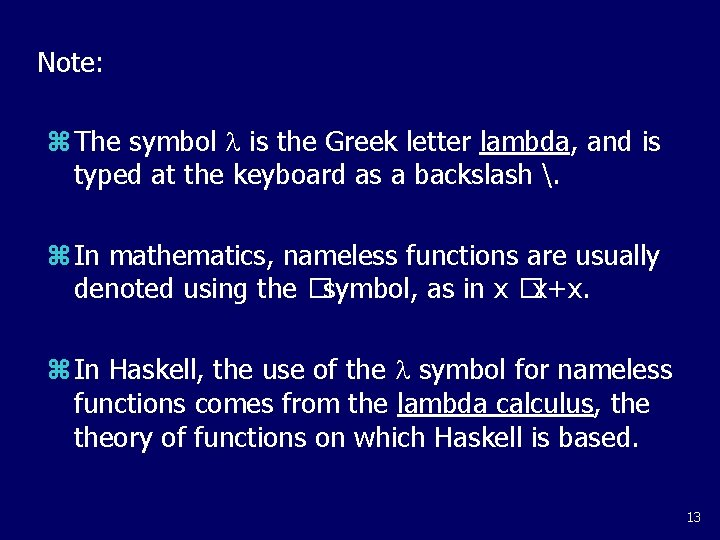Note: z The symbol is the Greek letter lambda, and is typed at the keyboard as a backslash . z In mathematics, nameless functions are usually denoted using the �symbol, as in x �x+x. z In Haskell, the use of the symbol for nameless functions comes from the lambda calculus, theory of functions on which Haskell is based. 13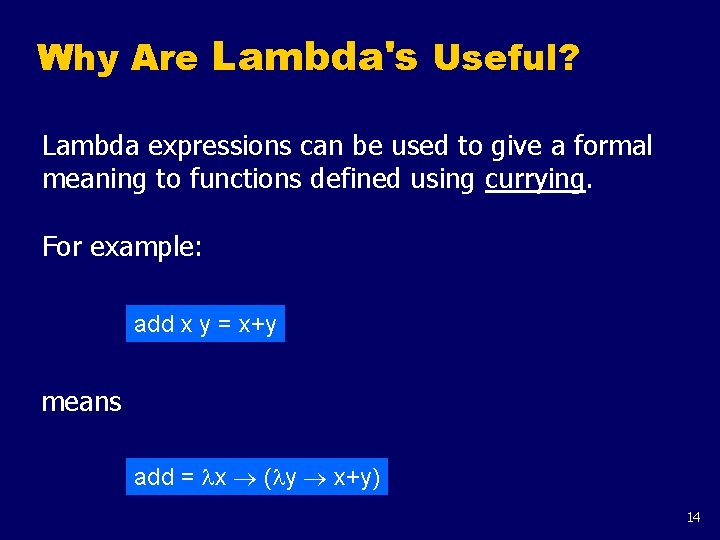Why Are Lambda's Useful? Lambda expressions can be used to give a formal meaning to functions defined using currying. For example: add x y = x+y means add = x ( y x+y) 14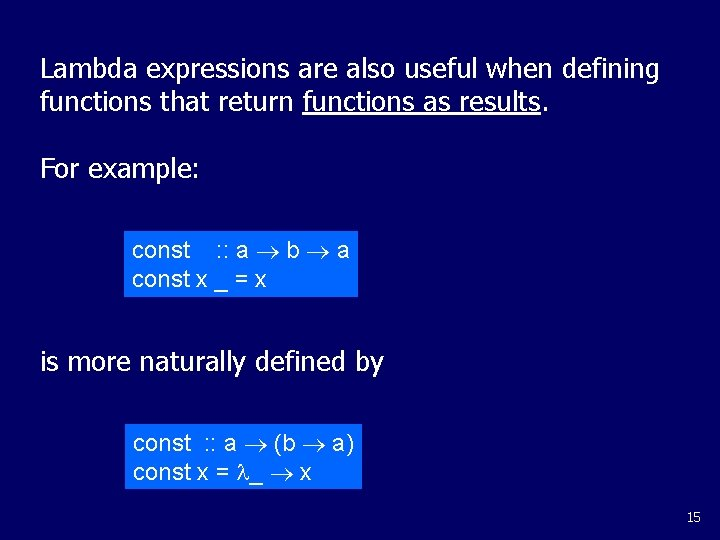Lambda expressions are also useful when defining functions that return functions as results. For example: const : : a b a const x _ = x is more naturally defined by const : : a (b a) const x = _ x 15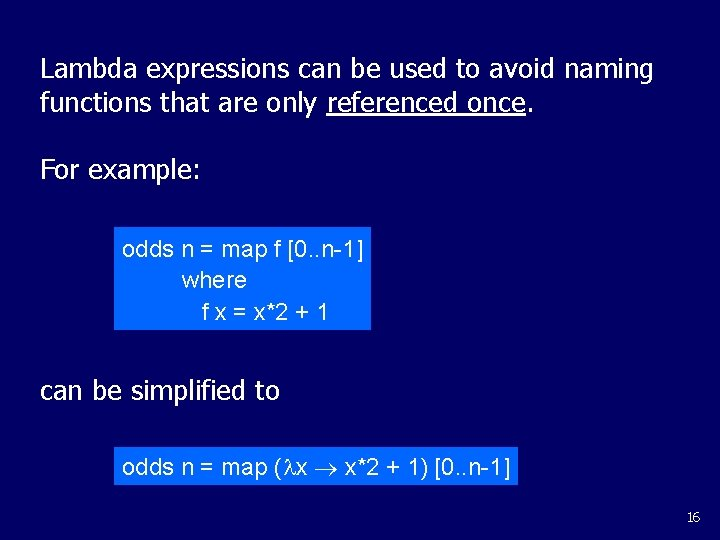Lambda expressions can be used to avoid naming functions that are only referenced once. For example: odds n = map f [0. . n-1] where f x = x*2 + 1 can be simplified to odds n = map ( x x*2 + 1) [0. . n-1] 16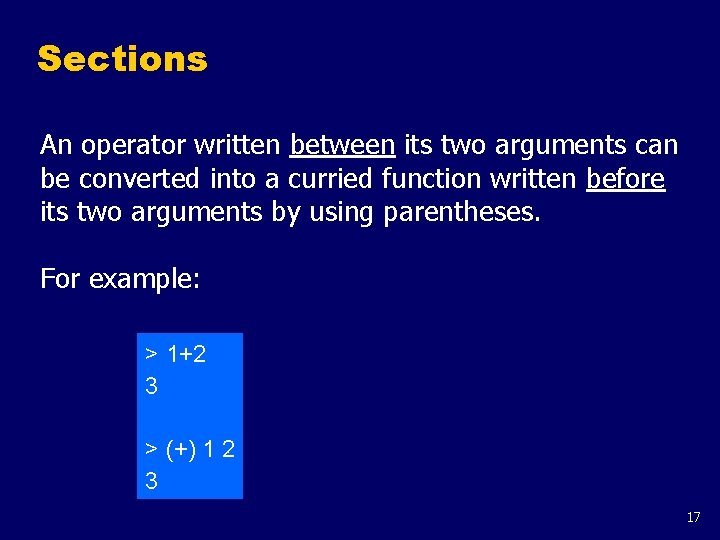Sections An operator written between its two arguments can be converted into a curried function written before its two arguments by using parentheses. For example: > 1+2 3 > (+) 1 2 3 17This convention also allows one of the arguments of the operator to be included in the parentheses. For example: > (1+) 2 3 > (+2) 1 3 In general, if is an operator then functions of the form ( ), (x ) and ( y) are called sections. 18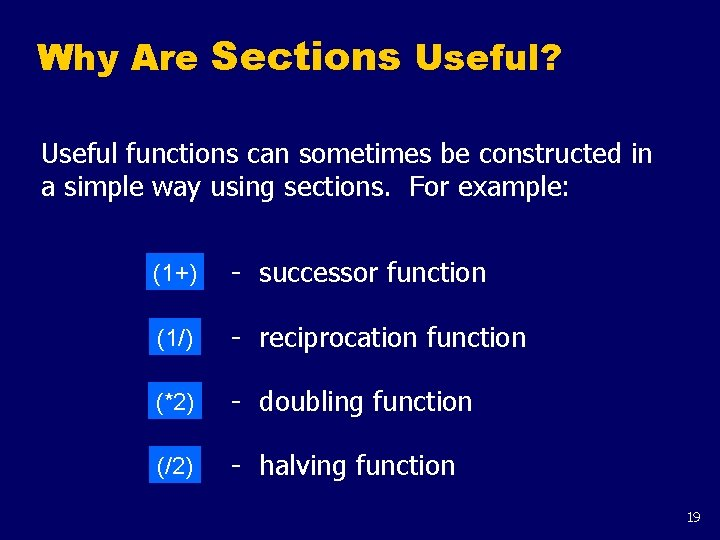Why Are Sections Useful? Useful functions can sometimes be constructed in a simple way using sections. For example: (1+) - successor function (1/) - reciprocation function (*2) - doubling function (/2) - halving function 19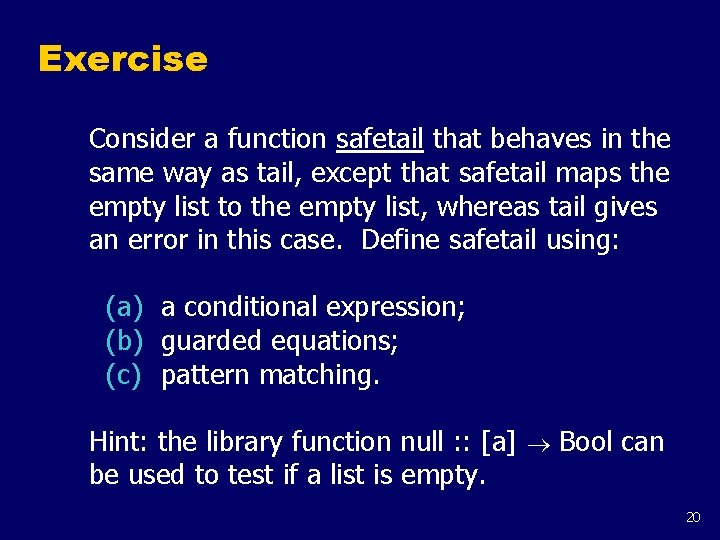Exercise Consider a function safetail that behaves in the same way as tail, except that safetail maps the empty list to the empty list, whereas tail gives an error in this case. Define safetail using: (a) a conditional expression; (b) guarded equations; (c) pattern matching. Hint: the library function null : : [a] Bool can be used to test if a list is empty. 20The Filter Function The higher-order library function filter selects every element from a list that satisfies a predicate. filter : : (a Bool) [a] For example: > filter even [1. . 10] [2, 4, 6, 8, 10] 21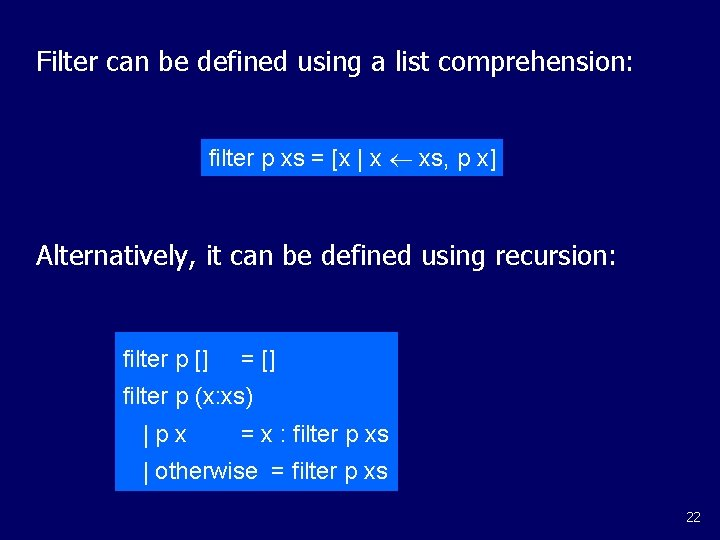Filter can be defined using a list comprehension: filter p xs = [x | x xs, p x] Alternatively, it can be defined using recursion: filter p [] = [] filter p (x: xs) |px = x : filter p xs | otherwise = filter p xs 22The Foldr Function A number of functions on lists can be defined using the following simple pattern of recursion: f [] = v f (x: xs) = x f xs f maps the empty list to some value v, and any non-empty list to some function applied to its head and f of its tail. 23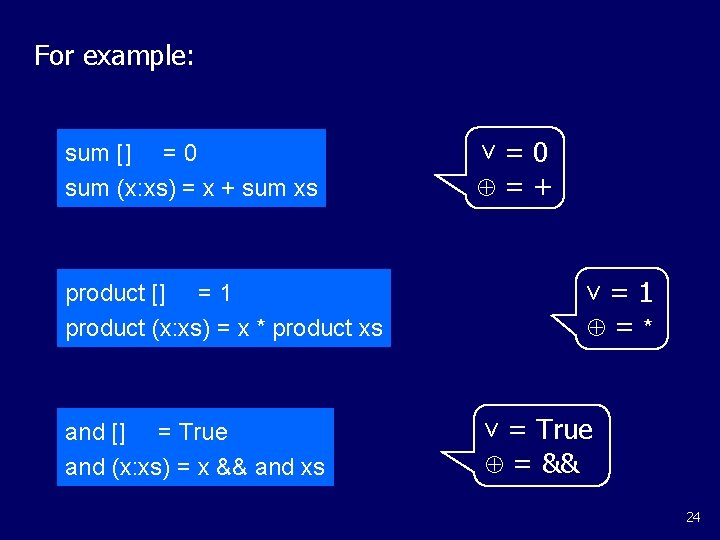For example: sum [] = 0 sum (x: xs) = x + sum xs v=0 =+ v=1 product [] = 1 product (x: xs) = x * product xs and [] = True and (x: xs) = x && and xs =* v = True = && 24The higher-order library function foldr (fold right) encapsulates this simple pattern of recursion, with the function and the value v as arguments. For example: sum = foldr (+) 0 product = foldr (*) 1 or and = foldr (||) False = foldr (&&) True 25Foldr itself can be defined using recursion: foldr : : (a b b) b [a] b foldr f v [] =v foldr f v (x: xs) = f x (foldr f v xs) However, it is best to think of foldr non-recursively, as simultaneously replacing each (: ) in a list by a given function, and [] by a given value. 26For example: = = sum [1, 2, 3] foldr (+) 0 (1: (2: (3: []))) 1+(2+(3+0)) 6 Replace each (: ) by (+) and [] by 0. 27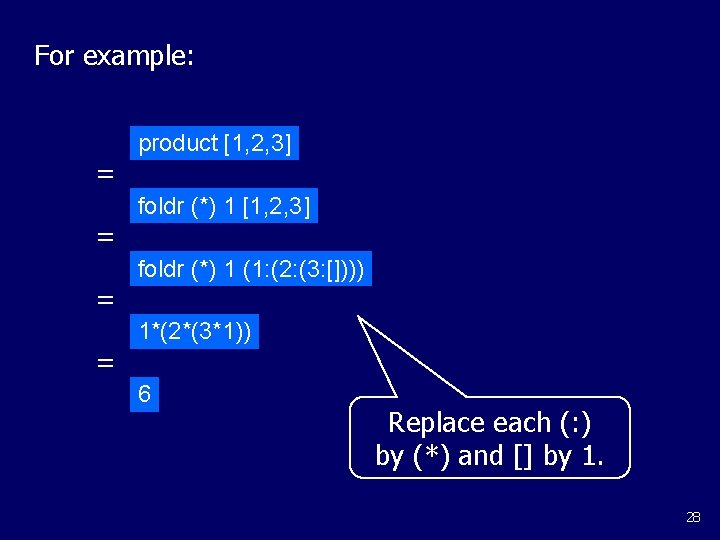For example: = = product [1, 2, 3] foldr (*) 1 (1: (2: (3: []))) 1*(2*(3*1)) 6 Replace each (: ) by (*) and [] by 1. 28Other Foldr Examples Even though foldr encapsulates a simple pattern of recursion, it can be used to define many more functions than might first be expected. Recall the length function: length : : [a] Int length [] =0 length (_: xs) = 1 + length xs 29For example: = = = length [1, 2, 3] length (1: (2: (3: []))) 1+(1+(1+0)) 3 Hence, we have: Replace each (: ) by _ n 1+n and [] by 0. length = foldr ( _ n 1+n) 0 30Now recall the reverse function: reverse [] = [] reverse (x: xs) = reverse xs ++ [x] For example: = = = reverse [1, 2, 3] Replace each (: ) by x xs ++ [x] and [] by []. reverse (1: (2: (3: []))) (([] ++ ) ++ ) ++  [3, 2, 1] 31Hence, we have: reverse = foldr ( x xs ++ [x]) [] Finally, we note that the append function (++) has a particularly compact definition using foldr: (++ ys) = foldr (: ) ys Replace each (: ) by (: ) and [] by ys. 32Why Is Foldr Useful? z Some recursive functions on lists, such as sum, are simpler to define using foldr. z Properties of functions defined using foldr can be proved using algebraic properties of foldr, such as fusion and the banana split rule. z Advanced program optimisations can be simpler if foldr is used in place of explicit recursion. 33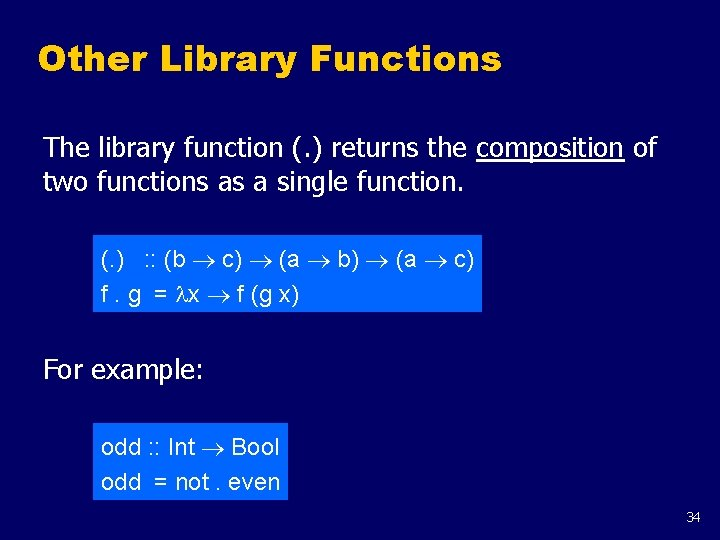Other Library Functions The library function (. ) returns the composition of two functions as a single function. (. ) : : (b c) (a b) (a c) f. g = x f (g x) For example: odd : : Int Bool odd = not. even 34The library function all decides if every element of a list satisfies a given predicate. all : : (a Bool) [a] Bool all p xs = and [p x | x xs] For example: > all even [2, 4, 6, 8, 10] True 35Dually, the library function any decides if at least one element of a list satisfies a predicate. any : : (a Bool) [a] Bool any p xs = or [p x | x xs] For example: > any is. Space "abc def" True 36The library function take. While selects elements from a list while a predicate holds of all the elements. take. While : : (a Bool) [a] take. While p [] = [] take. While p (x: xs) |px = x : take. While p xs | otherwise = [] For example: > take. While is. Alpha "abc def" "abc" 37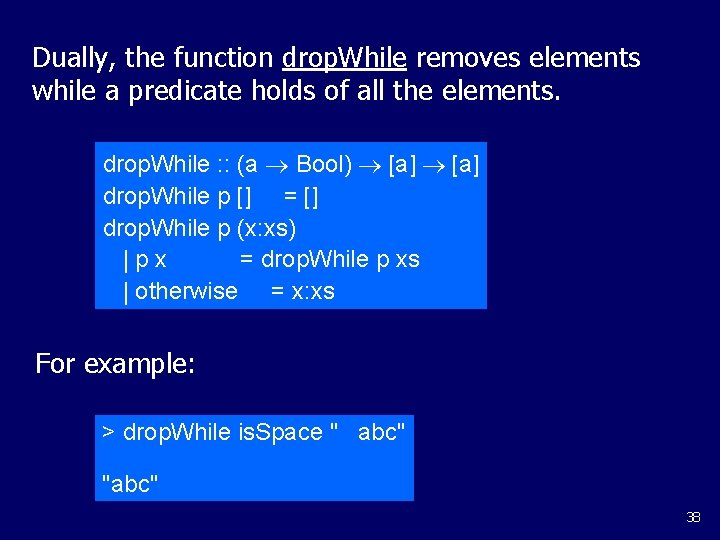Dually, the function drop. While removes elements while a predicate holds of all the elements. drop. While : : (a Bool) [a] drop. While p [] = [] drop. While p (x: xs) |px = drop. While p xs | otherwise = x: xs For example: > drop. While is. Space " abc" "abc" 38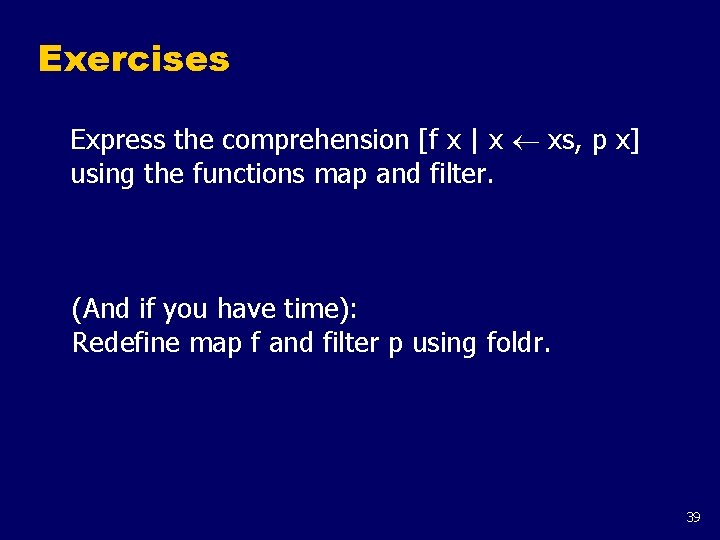Exercises Express the comprehension [f x | x xs, p x] using the functions map and filter. (And if you have time): Redefine map f and filter p using foldr. 39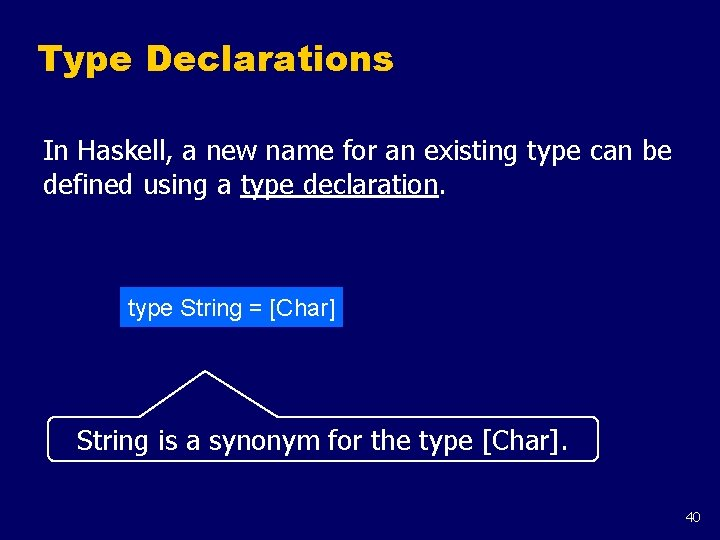Type Declarations In Haskell, a new name for an existing type can be defined using a type declaration. type String = [Char] String is a synonym for the type [Char]. 40Type declarations can be used to make other types easier to read. For example, given type Pos = (Int, Int) we can define: origin : : Pos = (0, 0) left : : Pos left (x, y) = (x-1, y) 41Like function definitions, type declarations can also have parameters. For example, given type Pair a = (a, a) we can define: mult : : Pair Int mult (m, n) = m*n copy : : a Pair a copy x = (x, x) 42Type declarations can be nested: type Pos = (Int, Int) type Trans = Pos However, they cannot be recursive: type Tree = (Int, [Tree]) 43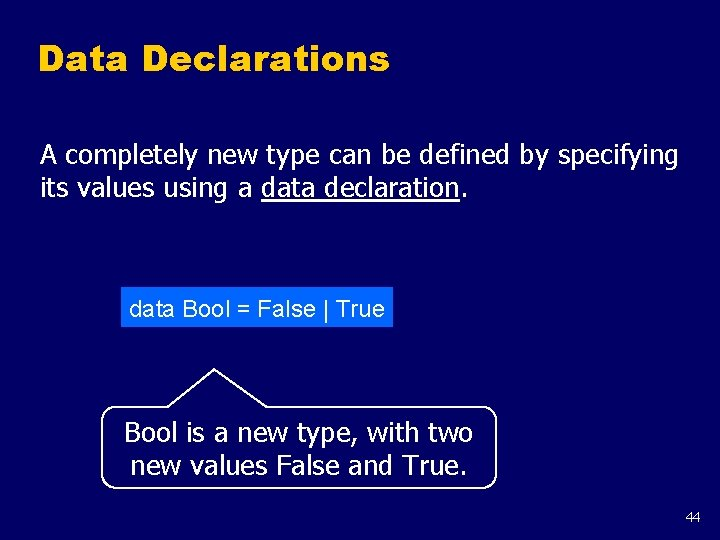Data Declarations A completely new type can be defined by specifying its values using a data declaration. data Bool = False | True Bool is a new type, with two new values False and True. 44Note: z The two values False and True are called the constructors for the type Bool. z Type and constructor names must begin with an upper-case letter. z Data declarations are similar to context free grammars. The former specifies the values of a type, the latter the sentences of a language. 45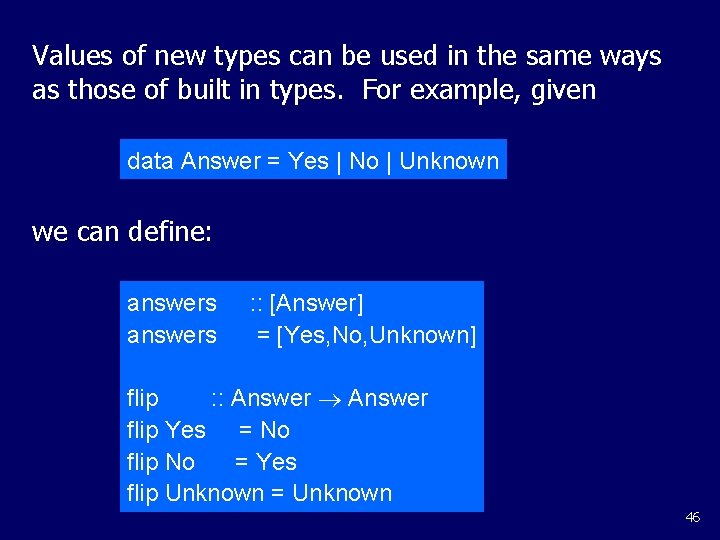Values of new types can be used in the same ways as those of built in types. For example, given data Answer = Yes | No | Unknown we can define: answers : : [Answer] = [Yes, No, Unknown] flip : : Answer flip Yes = No flip No = Yes flip Unknown = Unknown 46The constructors in a data declaration can also have parameters. For example, given data Shape = Circle Float | Rect Float we can define: square n : : Float Shape = Rect n n area : : Shape Float area (Circle r) = pi * r^2 area (Rect x y) = x * y 47Note: z Shape has values of the form Circle r where r is a float, and Rect x y where x and y are floats. z Circle and Rect can be viewed as functions that construct values of type Shape: Circle : : Float Shape Rect : : Float Shape 48Not surprisingly, data declarations themselves can also have parameters. For example, given data Maybe a = Nothing | Just a we can define: safediv : : Int Maybe Int safediv _ 0 = Nothing safediv m n = Just (m `div` n) safehead : : [a] Maybe a safehead [] = Nothing safehead xs = Just (head xs) 49Exercises Write a function safe. Second which takes a list and returns its second element (assuming it has one). Note: you’ll need the following: data Maybe a = Nothing | Just a safehead : : [a] Maybe a safehead [] = Nothing safehead xs = Just (head xs) 50